1575966342

# Ensemble, Deep Learning using LIME and SHAP

In this world of ever increasing data at a hyper pace, we use all kinds of complex ensemble and deep learning algorithms to achieve the highest possible accuracy. It’s sometimes magical how these models predict/classify/recognize/track on unknown data. And accomplishing this magic and more has been and would be the goal of intensive research and development in the data science community. But, around all this great work, a question arises, can we always trust this prediction/classification /recognition/tracking? A variety of reasons, like lack of data, imbalanced datasets, biased datasets etc. can impact the decision rendered by the learning models. Models are gaining traction for explainability. Financial institutions and law agencies, for example demand explanations and evidences (SR 11–7 and The FUTURE of AI Act) bolstering the output of these learning models.

I am going to demonstrate explainability on the decisions made by LightGBM and Keras models in classifying a transaction for fraudulence on the IEEE CIS dataset . I would use two state of the art open source explainability techniques in this article, namely LIME (https://github.com/marcotcr/lime) and SHAP (https://github.com/slundberg/shap) from these research papers (1, 2). I have saved another great open source explainability technique — AIX360 (https://github.com/IBM/AIX360) for my next post as it proposes 8 novel algorithms by itself for explainability.

I have borrowed the awesome feature engineering techniques on this dataset from here. Additionally, I have used feature scaling to even out the variability in the magnitude of feature values.

## LIME

Intuitively, an explanation is a local linear approximation of the model’s behaviour. While the model may be very complex globally, it is easier to approximate it around the vicinity of a particular instance. While treating the model as a black box, LIME perturbs the instance desired to explain and learn a sparse linear model around it, as an explanation. The figure below illustrates the intuition for this procedure. The model’s decision function is represented by the blue/pink background, and is clearly nonlinear. The bright red cross is the instance being explained (let’s call it X). We sample instances around X, and weight them according to their proximity to X (weight here is indicated by size). We then learn a linear model (dashed line) that approximates the model well in the vicinity of X, but not necessarily globally. For more information, read this paper, or take a look at this blog post (https://github.com/marcotcr/lime).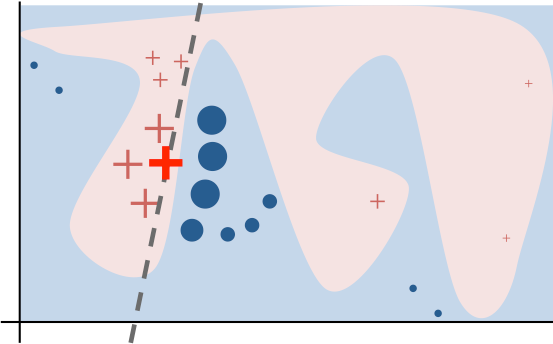LIME Technique (https://github.com/marcotcr/lime)

### Ensemble model — LightGBM

Below is my model configuration. I have got an auc score of 0.972832 for this model.

``````# Create training and validation sets
x_train, x_test, y_train, y_test = train_test_split(x, y, test_size=0.30, random_state=42)
# Train the model
parameters = {
'application': 'binary',
'objective': 'binary',
'metric': 'auc',
'is_unbalance': 'true',
'boosting': 'gbdt',
'num_leaves': 31,
'feature_fraction': 0.5,
'bagging_fraction': 0.5,
'bagging_freq': 20,
'learning_rate': 0.05,
'verbose': 0
}
model = lightgbm.train(parameters,
train_data,
valid_sets=test_data,
num_boost_round=5000,
early_stopping_rounds=100)
y_pred = model.predict(x_test)
``````

This the classification report of the above model.

``````from sklearn.metrics import classification_report
print(classification_report(y_test, y_pred_bool))
``````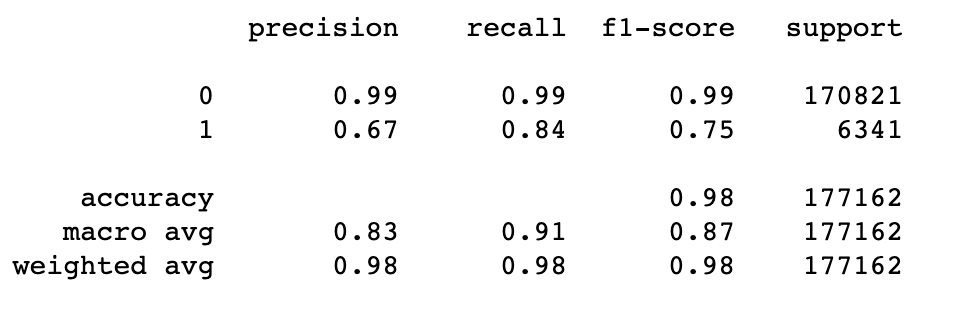Before, I explore the formal LIME and SHAP explainability techniques to explain the model classification results, I thought why not use LightGBM’s inbuilt ‘feature importance’ function to visually understand the 20 most important features which helped the model lean towards a particular classification.

``````feature_imp= pd.DataFrame({'Value':model.feature_importance(),'Feature':X.columns})
plt.figure(figsize=(40, 20))
sns.set(font_scale = 5)
sns.barplot(x="Value", y="Feature", data=feature_imp.sort_values(by="Value", ascending=False)[0:20])
plt.title('LightGBM Features (avg over folds)')
plt.tight_layout()
plt.savefig('lgbm_importances-01.png')
plt.show()
``````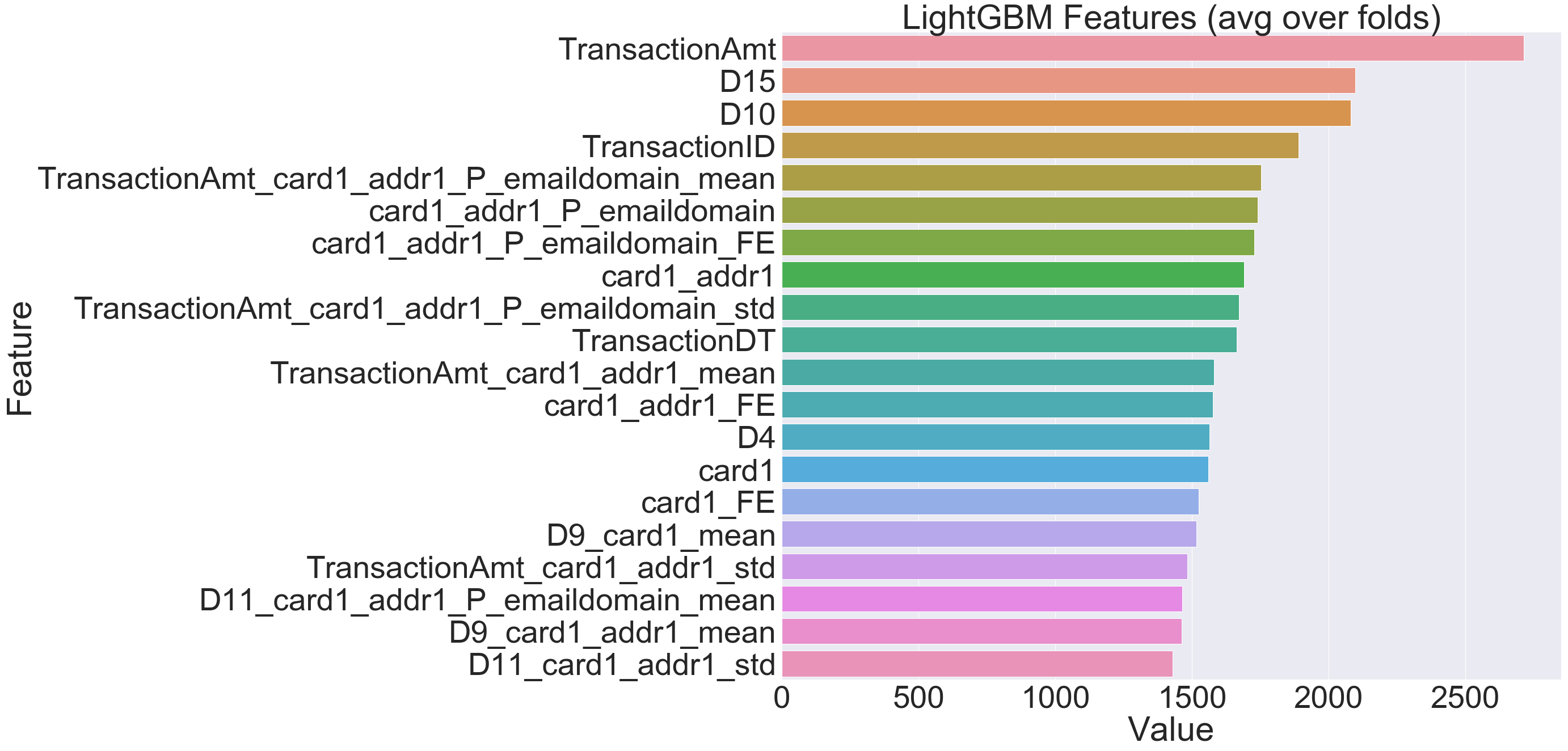Having seen the top 20 crucial features enabling the model, let us dive into explaining these decisions through few amazing open source python libraries, namely LIME (https://github.com/marcotcr/lime) and SHAP (https://github.com/slundberg/shap).

The code for using LIME to explain the decisions made by model is simple and takes few lines.

``````def prob(data):
return np.array(list(zip(1-model.predict(data),model.predict(data))))
import lime
import lime.lime_tabular
explainer = lime.lime_tabular.LimeTabularExplainer(new_df[list(X.columns)].astype(int).values,
mode='classification',training_labels=new_df['isFraud'],feature_names=list(X.columns))
``````

I would use this explainer to explain any random instance as follows:

``````i = 2
exp = explainer.explain_instance(new_df.loc[i,list(X.columns)].astype(int).values, prob, num_features=10)
##visualize the result
exp.show_in_notebook(show_table=True)
``````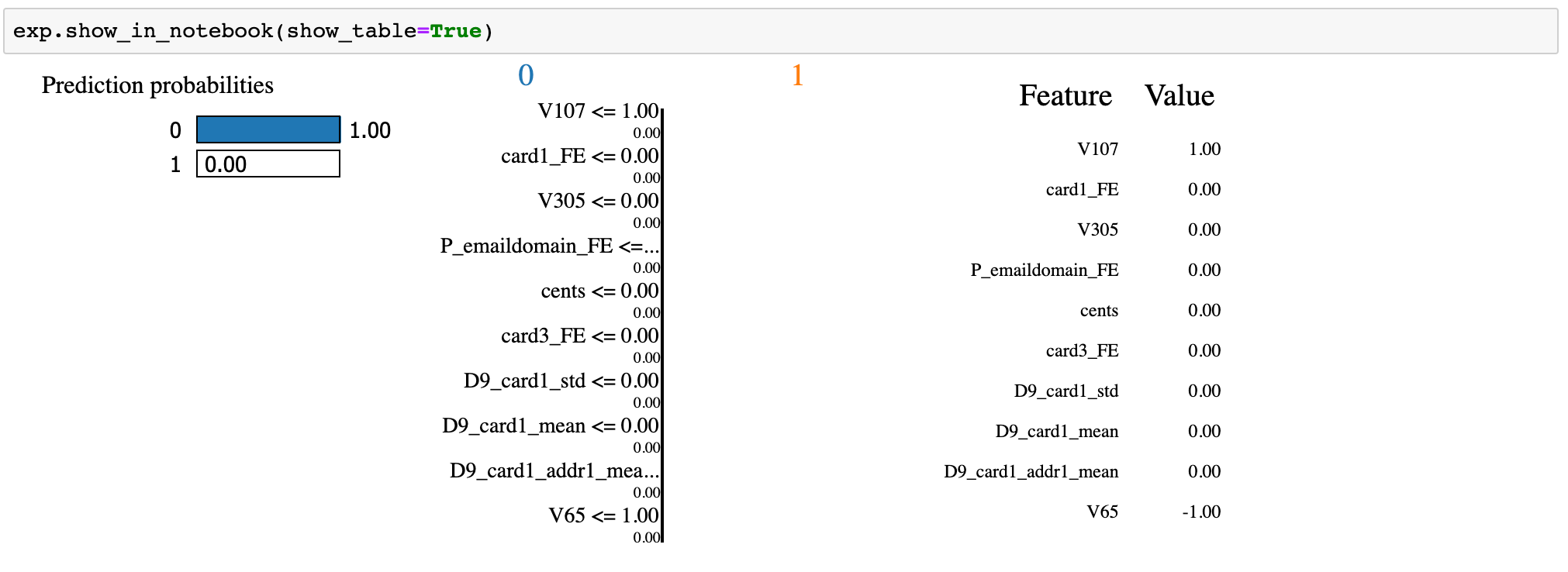## Deep Learning model — Keras (tensorflow)

I have attempted to explain the decisions of my neural network (Keras) model as below. The model is configured as follows.

``````classifier = Sequential()
#First Hidden Layer
#Second  Hidden Laye
#Output Layer
``````

This the classification report of the above model.

``````from sklearn.metrics import classification_report
y_pred=classifier.predict(X_test, batch_size=64, verbose=1)
y_pred =(y_pred>0.5)
y_pred_bool = np.argmax(y_pred, axis=1)
print(classification_report(Y_test, y_pred_bool))
``````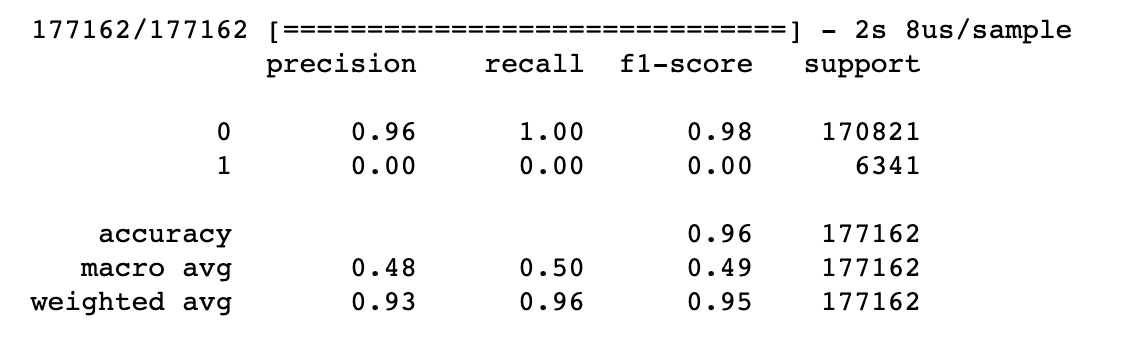Following is the code for LIME explainer for the results of the above Keras model.

``````def prob(data):
print(data.shape)
y_pred=classifier.predict(data).reshape(-1, 1)
y_pred =(y_pred>0.5)
print(np.array(list(zip(1-y_pred.reshape(data.shape),y_pred.reshape(data.shape)))))
return np.hstack((1-y_pred,y_pred))
import lime
import lime.lime_tabular
explainer = lime.lime_tabular.LimeTabularExplainer(X[list(X.columns)].astype(int).values,
mode='classification',training_labels=new_df['isFraud'],feature_names=list(X.columns))
``````

I would use the above explainer to explain any random instance as below.

``````i = 19
exp = explainer.explain_instance(X.loc[i,X.columns].astype(int).values, prob, num_features=5)
``````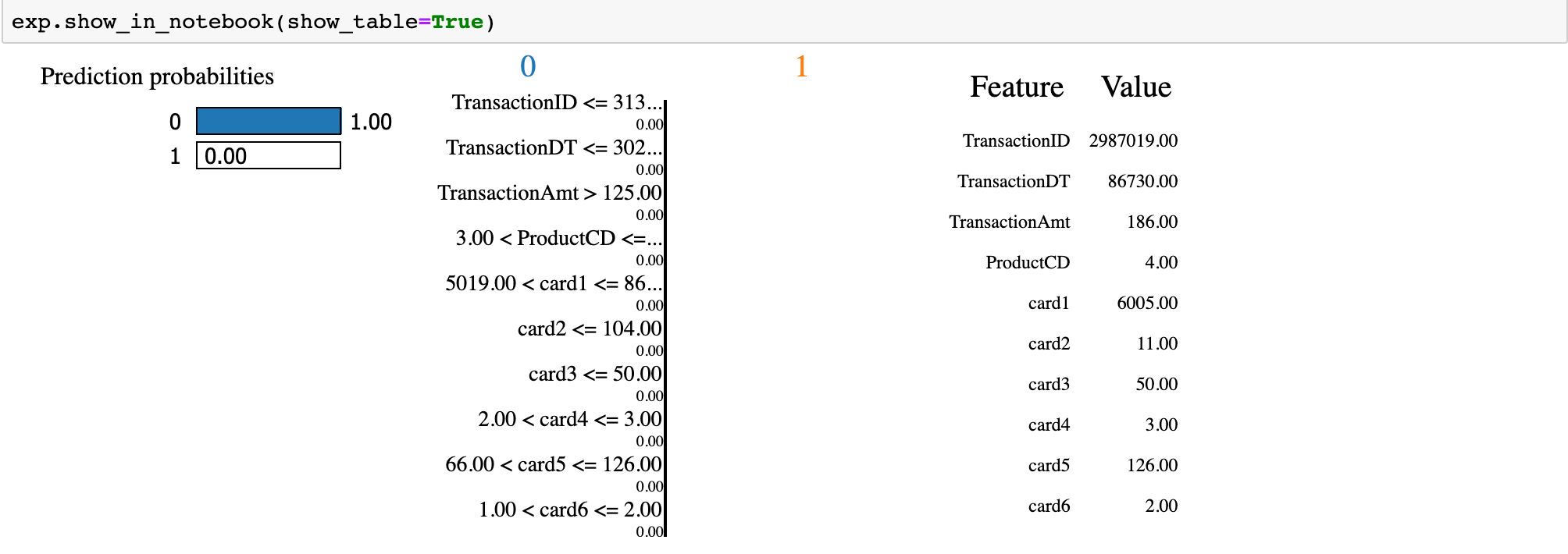The beauty of SHAP (SHapley Additive exPlanations) lies in the fact that it unifies all available frameworks for interpreting predictions. SHAP assigns each feature an importance value for a particular prediction. Its novel components include: (1) the identification of a new class of additive feature importance measures, and (2) theoretical results showing there is a unique solution in this class with a set of desirable properties. The new class unifies six existing methods, notable because several recent methods in the class lack the proposed desirable properties. Based on insights from this unification, it presents new methods that show improved computational performance and/or better consistency with human intuition than previous approaches.

## Ensemble model — LightGBM

``````import shap
# load JS visualization code to notebook
shap.initjs()
# explain the model's predictions using SHAP values
# (this syntax works for LightGBM, CatBoost, scikit-learn and spark models)
explainer = shap.TreeExplainer(model)
shap_values = explainer.shap_values(X)
# visualize the first prediction's explanation (use matplotlib=True to avoid Javascript)
shap.force_plot(explainer.expected_value, shap_values[0,:], X.iloc[0,:])

``````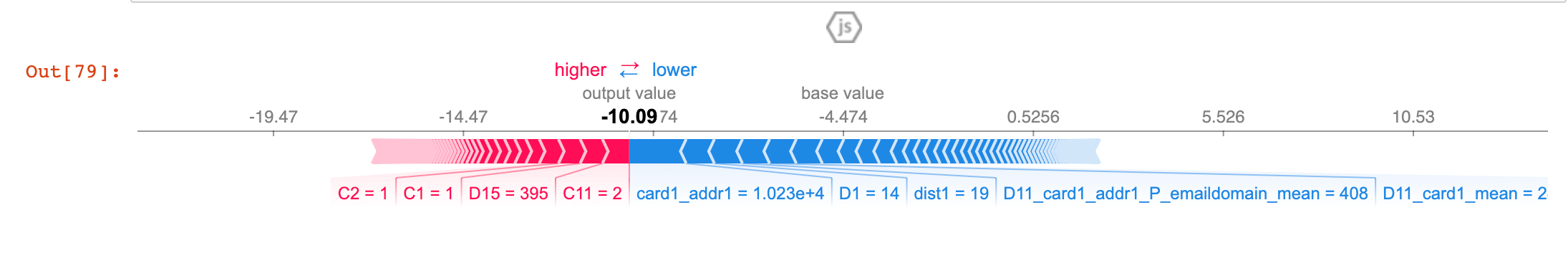The above explanation shows features each contributing to push the model output from the base value (the average model output over the training dataset we passed) to the model output. Features pushing the prediction higher are shown in red, those pushing the prediction lower are in blue (these force plots were introduced in the Nature BME paper). If we take many explanations such as the one shown above, rotate them 90 degrees, and then stack them horizontally, we can see explanations for an entire dataset .

``````shap.dependence_plot("TransactionAmt", shap_values, X)
``````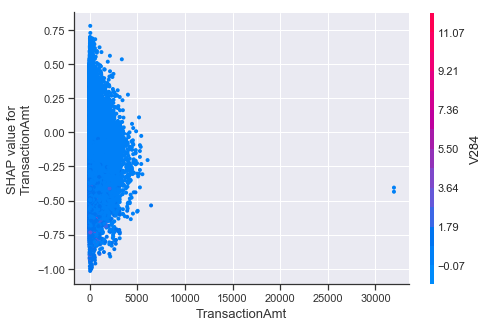To understand how a single feature affects the output of the model we can plot the SHAP value of that feature vs. the value of the feature for all the examples in a dataset. Since SHAP values represent a feature’s responsibility for a change in the model output, the plot below represents the change in predicted house price as TransactionAmt (the amount in the transaction) changes. Vertical dispersion at a single value of TransactionAmt represents interaction effects with other features. To help reveal these interactions `dependence_plot` automatically selects another feature for coloring. In this case coloring by V284 highlights that the average number of TransactionAmt has less impact on fraud probability for cases with a high V284 value.

``````# summarize the effects of all the features
shap.summary_plot(shap_values, X)
``````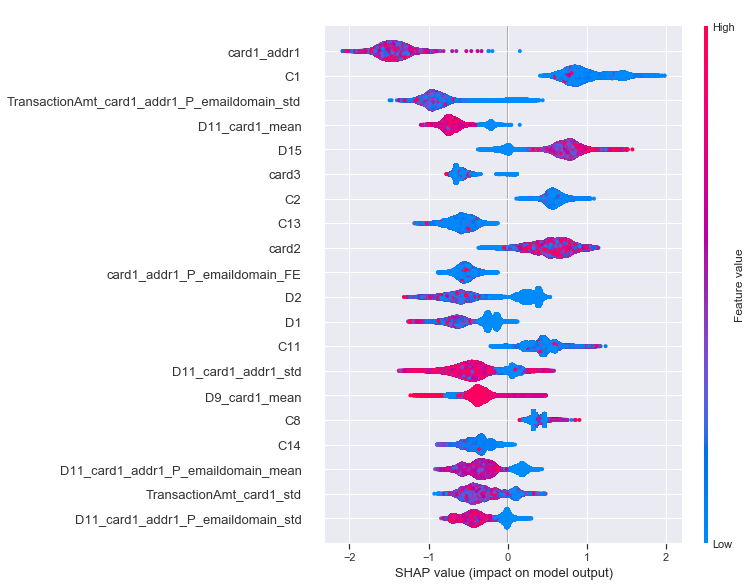To get an overview of which features are most important for a model we can plot the SHAP values of every feature for every sample. The plot below sorts features by the sum of SHAP value magnitudes over all samples, and uses SHAP values to show the distribution of the impacts each feature has on the model output. The color represents the feature value (red high, blue low). This reveals for example that a card holder address has a high impact on its predicted fraudulent status.

``````shap.summary_plot(shap_values, X, plot_type="bar")
``````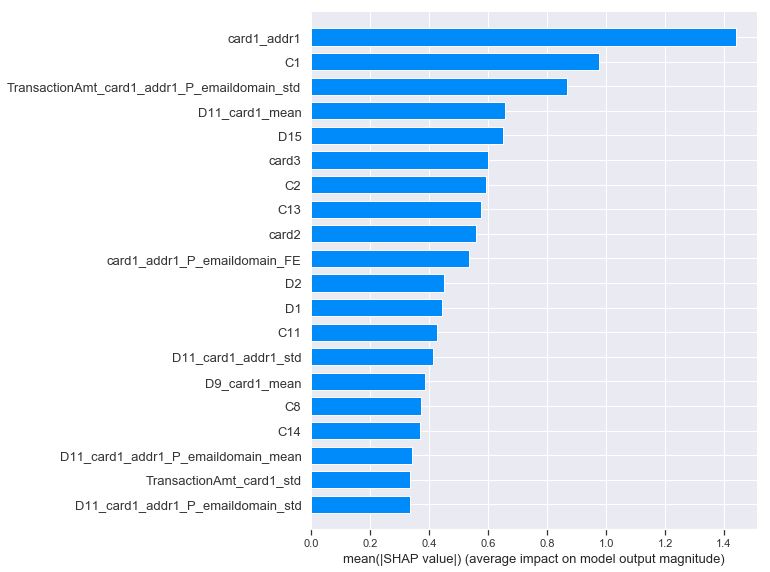We can also just take the mean absolute value of the SHAP values for each feature to get a standard bar plot

## Deep Learning model — Keras (tensorflow)

In a similar way as LightGBM, we can use SHAP on deep learning as below; but this time we would use the keras compatible DeepExplainer instead of TreeExplainer.

``````import shap
import tensorflow.keras.backend
background = X_train[np.random.choice(X_train.shape, 100, replace=False)]
# we use the first 100 training examples as our background dataset to integrate over
explainer = shap.DeepExplainer(classifier,  background)
``````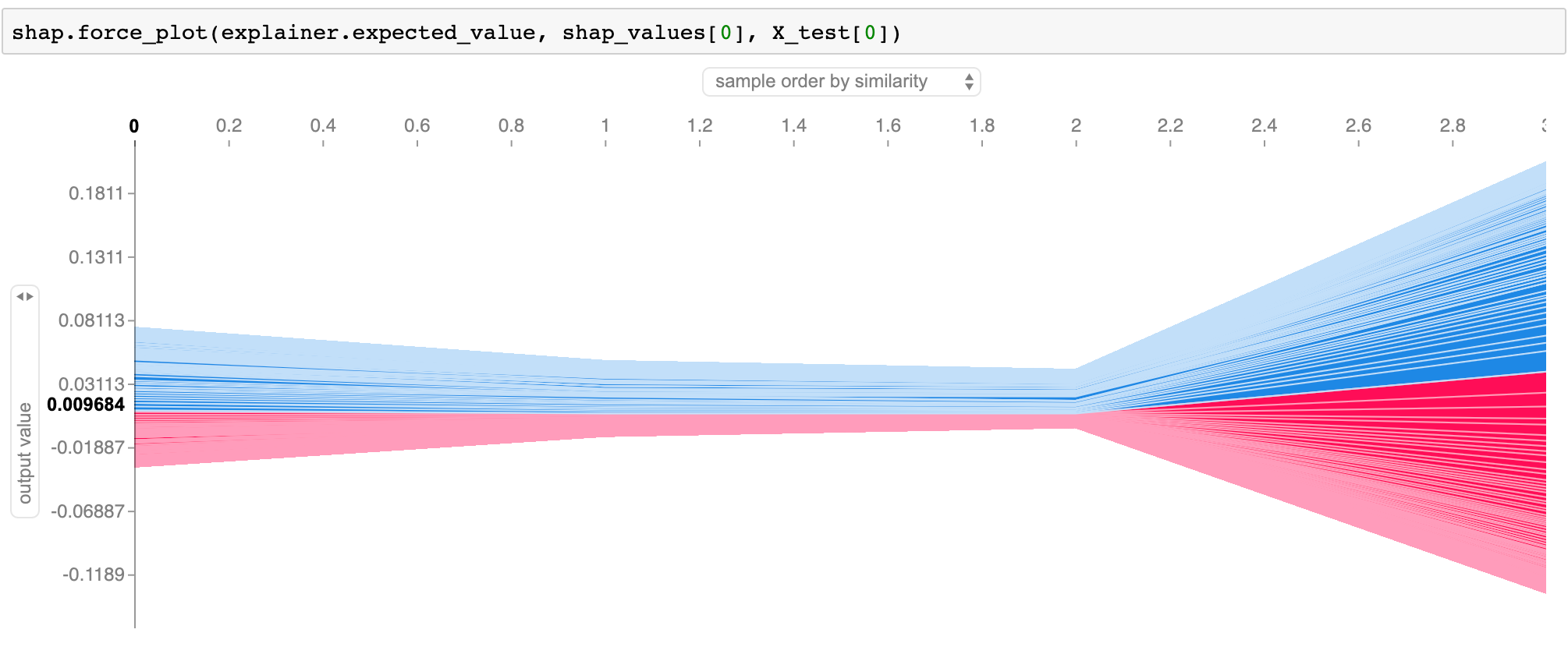Features pushing the prediction higher are shown in red, those pushing the prediction lower are in blue (these force plots are introduced in the Nature BME paper).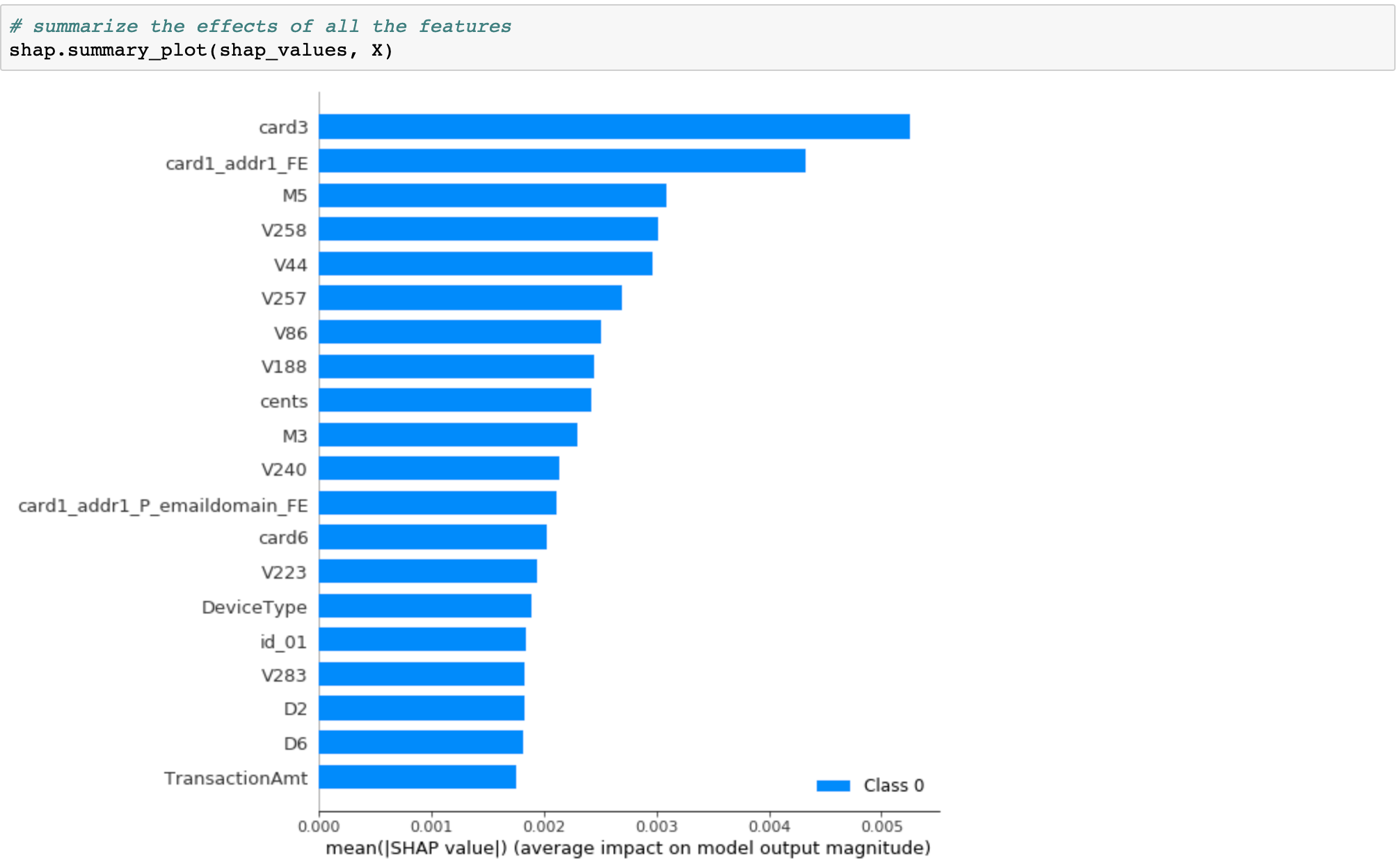We can also just take the mean absolute value of the SHAP values for each feature to get a standard bar plot

## Differences between SHAP and LIME

LIME creates a surrogate model locally around the unit who’s prediction you wish to understand. Thus it is inherently local. Shapely values ‘decompose’ the final prediction into the contribution of each attribute — this is what some mean by ‘consistent’ (the values add up to the actual prediction of the true model, this is not something you get with LIME). But to actually get the shapely values there is some decision that must be made about what to do/how to handle the values of the attributes ‘left out’, this is how the values are arrived at. In this decision there is some choice which could change the interpretation. If I ‘leave out’ an attribute do I average all the possibilities? Do choose some ‘baseline’? So Shapely actually tells you, in an additive way, how you got your score, but there is some choice about the ‘starting point’ (i.e. the decision about omitted attributes). LIME simply tells you, in a local sense, what is the most important attribute around the data point of interest.

In production, we can use the following architecture to share model explainability with the stakeholders.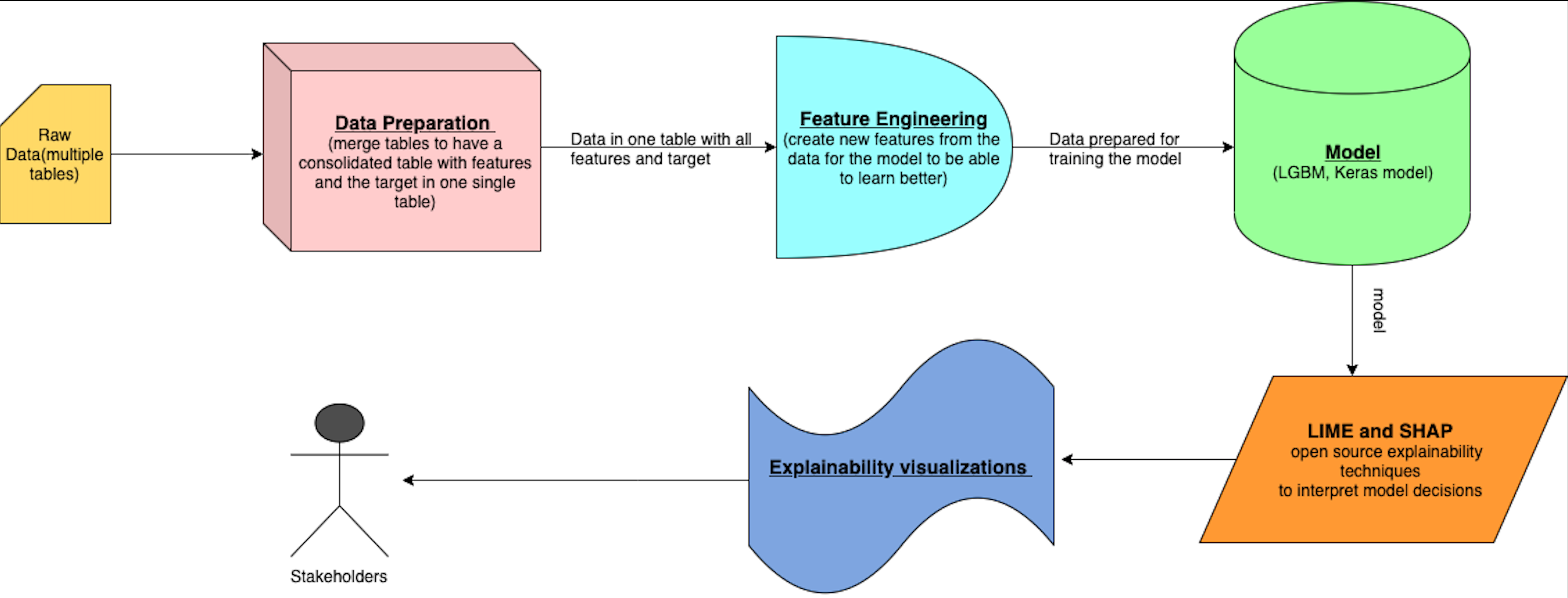#deep-learning #machine-learning #tensorflow

## Buddha Community1618317562

## Top Deep Learning Development Services | Hire Deep Learning Developer

We at Inexture, strategically work on every project we are associated with. We propose a robust set of AI, ML, and DL consulting services. Our virtuoso team of data scientists and developers meticulously work on every project and add a personalized touch to it. Because we keep our clientele aware of everything being done associated with their project so there’s a sense of transparency being maintained. Leverage our services for your next AI project for end-to-end optimum services.

#deep learning development #deep learning framework #deep learning expert #deep learning ai #deep learning services1603735200

## Top 10 Deep Learning Sessions To Look Forward To At DVDC 2020

The Deep Learning DevCon 2020, DLDC 2020, has exciting talks and sessions around the latest developments in the field of deep learning, that will not only be interesting for professionals of this field but also for the enthusiasts who are willing to make a career in the field of deep learning. The two-day conference scheduled for 29th and 30th October will host paper presentations, tech talks, workshops that will uncover some interesting developments as well as the latest research and advancement of this area. Further to this, with deep learning gaining massive traction, this conference will highlight some fascinating use cases across the world.

Here are ten interesting talks and sessions of DLDC 2020 that one should definitely attend:

#### Adversarial Robustness in Deep Learning

By Dipanjan Sarkar

**About: **Adversarial Robustness in Deep Learning is a session presented by Dipanjan Sarkar, a Data Science Lead at Applied Materials, as well as a Google Developer Expert in Machine Learning. In this session, he will focus on the adversarial robustness in the field of deep learning, where he talks about its importance, different types of adversarial attacks, and will showcase some ways to train the neural networks with adversarial realisation. Considering abstract deep learning has brought us tremendous achievements in the fields of computer vision and natural language processing, this talk will be really interesting for people working in this area. With this session, the attendees will have a comprehensive understanding of adversarial perturbations in the field of deep learning and ways to deal with them with common recipes.

Read an interview with Dipanjan Sarkar.

#### Imbalance Handling with Combination of Deep Variational Autoencoder and NEATER

By Divye Singh

**About: **Imbalance Handling with Combination of Deep Variational Autoencoder and NEATER is a paper presentation by Divye Singh, who has a masters in technology degree in Mathematical Modeling and Simulation and has the interest to research in the field of artificial intelligence, learning-based systems, machine learning, etc. In this paper presentation, he will talk about the common problem of class imbalance in medical diagnosis and anomaly detection, and how the problem can be solved with a deep learning framework. The talk focuses on the paper, where he has proposed a synergistic over-sampling method generating informative synthetic minority class data by filtering the noise from the over-sampled examples. Further, he will also showcase the experimental results on several real-life imbalanced datasets to prove the effectiveness of the proposed method for binary classification problems.

#### Default Rate Prediction Models for Self-Employment in Korea using Ridge, Random Forest & Deep Neural Network

By Dongsuk Hong

About: This is a paper presentation given by Dongsuk Hong, who is a PhD in Computer Science, and works in the big data centre of Korea Credit Information Services. This talk will introduce the attendees with machine learning and deep learning models for predicting self-employment default rates using credit information. He will talk about the study, where the DNN model is implemented for two purposes — a sub-model for the selection of credit information variables; and works for cascading to the final model that predicts default rates. Hong’s main research area is data analysis of credit information, where she is particularly interested in evaluating the performance of prediction models based on machine learning and deep learning. This talk will be interesting for the deep learning practitioners who are willing to make a career in this field.

#opinions #attend dldc 2020 #deep learning #deep learning sessions #deep learning talks #dldc 2020 #top deep learning sessions at dldc 2020 #top deep learning talks at dldc 20201593529260

## Few Shot Learning — A Case Study (2)

In the previous blog, we looked into the fact why Few Shot Learning is essential and what are the applications of it. In this article, I will be explaining the Relation Network for Few-Shot Classification (especially for image classification) in the simplest way possible. Moreover, I will be analyzing the Relation Network in terms of:

1. Effectiveness of different architectures such as Residual and Inception Networks
2. Effects of transfer learning via using pre-trained classifier on ImageNet dataset

Moreover, effectiveness will be evaluated on the accuracy, time required for training, and the number of required training parameters.

Please watch the GitHub repository to check out the implementations and keep updated with further experiments.

# Introduction to Few-Shot Classification

In few shot classification, our objective is to design a method which can identify any object images by analyzing few sample images of the same class. Let’s the take one example to understand this. Suppose Bob has a client project to design a 5 class classifier, where 5 classes can be anything and these 5 classes can even change with time. As discussed in previous blog, collecting the huge amount of data is very tedious task. Hence, in such cases, Bob will rely upon few shot classification methods where his client can give few set of example images for each classes and after that his system can perform classification young these examples with or without the need of additional training.

In general, in few shot classification four terminologies (N way, K shot, support set, and query set) are used.

1. N way: It means that there will be total N classes which we will be using for training/testing, like 5 classes in above example.
2. K shot: Here, K means we have only K example images available for each classes during training/testing.
3. Support set: It represents a collection of all available K examples images from each classes. Therefore, in support set we have total N*K images.
4. Query set: This set will have all the images for which we want to predict the respective classes.

At this point, someone new to this concept will have doubt regarding the need of support and query set. So, let’s understand it intuitively. Whenever humans sees any object for the first time, we get the rough idea about that object. Now, in future if we see the same object second time then we will compare it with the image stored in memory from the when we see it for the first time. This applied to all of our surroundings things whether we see, read, or hear. Similarly, to recognise new images from query set, we will provide our model a set of examples i.e., support set to compare.

And this is the basic concept behind Relation Network as well. In next sections, I will be giving the rough idea behind Relation Network and I will be performing different experiments on 102-flower dataset.

The Core idea behind Relation Network is to learn the generalized image representations for each classes using support set such that we can compare lower dimensional representation of query images with each of the class representations. And based on this comparison decide the class of each query images. Relation Network has two modules which allows us to perform above two tasks:

1. Embedding module: This module will extract the required underlying representations from each input images irrespective of the their classes.
2. Relation Module: This module will score the relation of embedding of query image with each class embedding.

Training/Testing procedure:

We can define the whole procedure in just 5 steps.

1. Use the support set and get underlying representations of each images using embedding module.
2. Take the average of between each class images and get the single underlying representation for each class.
3. Then get the embedding for each query images and concatenate them with each class’ embedding.
4. Use the relation module to get the scores. And class with highest score will be the label of respective query image.
5. [Only during training] Use MSE loss functions to train both (embedding + relation) modules.Few things to know during the training is that we will use only images from the set of selective class, and during the testing, we will be using images from unseen classes. For example, from the 102-flower dataset, we will use 50% classes for training, and rest will be used for validation and testing. Moreover, in each episode, we will randomly select 5 classes to create the support and query set and follow the above 5 steps.

That is all need to know about the implementation point of view. Although the whole process is simple and easy to understand, I’ll recommend reading the published research paper, Learning to Compare: Relation Network for Few-Shot Learning, for better understanding.

#deep-learning #few-shot-learning #computer-vision #machine-learning #deep learning #deep learning1595573880

## Deep Reinforcement Learning for Video Games Made Easy

In this post, we will investigate how easily we can train a Deep Q-Network (DQN) agent (Mnih et al., 2015) for Atari 2600 games using the Google reinforcement learning library Dopamine. While many RL libraries exist, this library is specifically designed with four essential features in mind:

• Easy experimentation
• Flexible development
• Compact and reliable
• Reproducible

_We believe these principles makes __Dopamine _one of the best RL learning environment available today. Additionally, we even got the library to work on Windows, which we think is quite a feat!

In my view, the visualization of any trained RL agent is an absolute must in reinforcement learning! Therefore, we will (of course) include this for our own trained agent at the very end!

We will go through all the pieces of code required (which is** minimal compared to other libraries**), but you can also find all scripts needed in the following Github repo.

# 1. Brief Introduction to Reinforcement Learning and Deep Q-Learning

The general premise of deep reinforcement learning is to

“derive efficient representations of the environment from high-dimensional sensory inputs, and use these to generalize past experience to new situations.”

• Mnih et al. (2015)

As stated earlier, we will implement the DQN model by Deepmind, which only uses raw pixels and game score as input. The raw pixels are processed using convolutional neural networks similar to image classification. The primary difference lies in the objective function, which for the DQN agent is called the optimal action-value function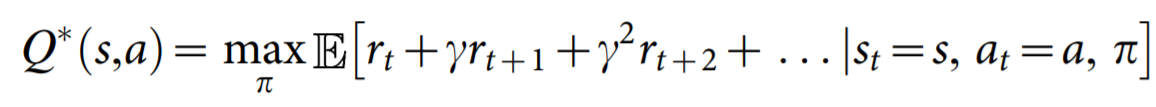where_ rₜ is the maximum sum of rewards at time t discounted by γ, obtained using a behavior policy π = P(a_∣_s)_ for each observation-action pair.

There are relatively many details to Deep Q-Learning, such as Experience Replay (Lin, 1993) and an _iterative update rule. _Thus, we refer the reader to the original paper for an excellent walk-through of the mathematical details.

One key benefit of DQN compared to previous approaches at the time (2015) was the ability to outperform existing methods for Atari 2600 games using the same set of hyperparameters and only pixel values and game score as input, clearly a tremendous achievement.

# 2. Installation

This post does not include instructions for installing Tensorflow, but we do want to stress that you can use both the CPU and GPU versions.

Nevertheless, assuming you are using `Python 3.7.x`, these are the libraries you need to install (which can all be installed via `pip`):

``````tensorflow-gpu=1.15   (or tensorflow==1.15  for CPU version)
cmake
dopamine-rl
atari-py
matplotlib
pygame
seaborn
pandas
``````

#reinforcement-learning #q-learning #games #machine-learning #deep-learning #deep learning1596178620

## Learn Transfer Learning for Deep Learning by implementing the project.

Project walkthrough on Convolution neural networks using transfer learning

From 2 years of my master’s degree, I found that the best way to learn concepts is by doing the projects. Let’s start implementing or in other words learning.

## Problem Statement

Take an image as input and return a corresponding dog breed from 133 dog breed categories. If a dog is detected in the image, it will provide an estimate of the dog’s breed. If a human is detected, it will give an estimate of the dog breed that is most resembling the human face. If there’s no human or dog present in the image, we simply print an error.

Let’s break this problem into steps

1. Detect Humans
2. Detect Dogs
3. Classify Dog breeds

For all these steps, we use pre-trained models.

Pre-trained models are saved models that were trained on a huge image-classification task such as Imagenet. If these datasets are huge and generalized enough, the saved weights can be used for multiple image detection task to get a high accuracy quickly.

## Detect Humans

For detecting humans, OpenCV provides many pre-trained face detectors. We use OpenCV’s implementation of Haar feature-based cascade classifiers to detect human faces in images.

``````### returns "True" if face is detected in image stored at img_path
def face_detector(img_path):
gray = cv2.cvtColor(img, cv2.COLOR_BGR2GRAY)
return len(faces) > 0
``````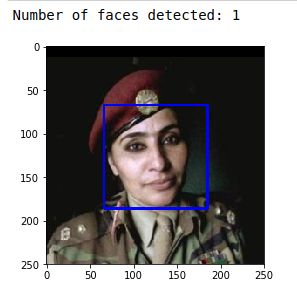## Detect Dogs

For detecting dogs, we use a pre-trained ResNet-50 model to detect dogs in images, along with weights that have been trained on ImageNet, a very large, very popular dataset used for image classification and other vision tasks.

``````from keras.applications.resnet50 import ResNet50

### define ResNet50 model
ResNet50_model_detector = ResNet50(weights='imagenet')
### returns "True" if a dog is detected
def dog_detector(img_path):
prediction = ResNet50_predict_labels(img_path)
return ((prediction <= 268) & (prediction >= 151))
``````

## Classify Dog Breeds

For classifying Dog breeds, we use transfer learning

Transfer learning involves taking a pre-trained neural network and adapting the neural network to a new, different data set.

To illustrate the power of transfer learning. Initially, we will train a simple CNN with the following architecture: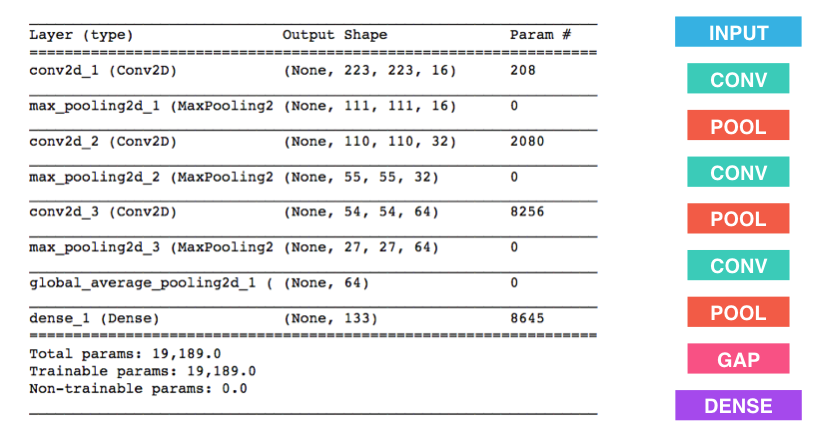Train it for 20 epochs, and it gives a test accuracy of just 3% which is better than a random guess from 133 categories. But with more epochs, we can increase accuracy, but it takes up a lot of training time.

To reduce training time without sacrificing accuracy, we will train the CNN model using transfer learning.

#data-science #transfer-learning #project-based-learning #cnn #deep-learning #deep learning